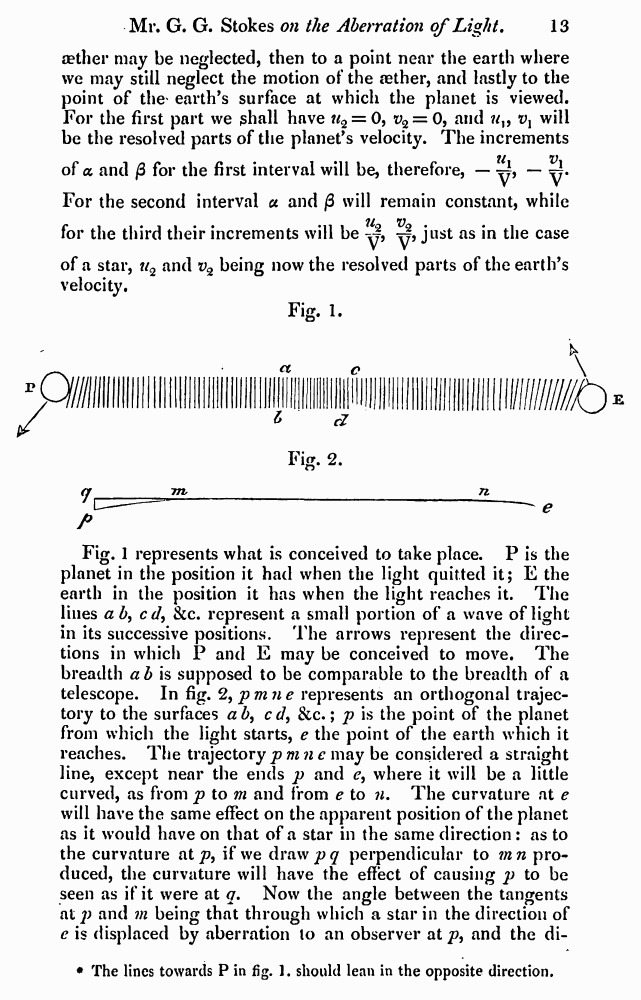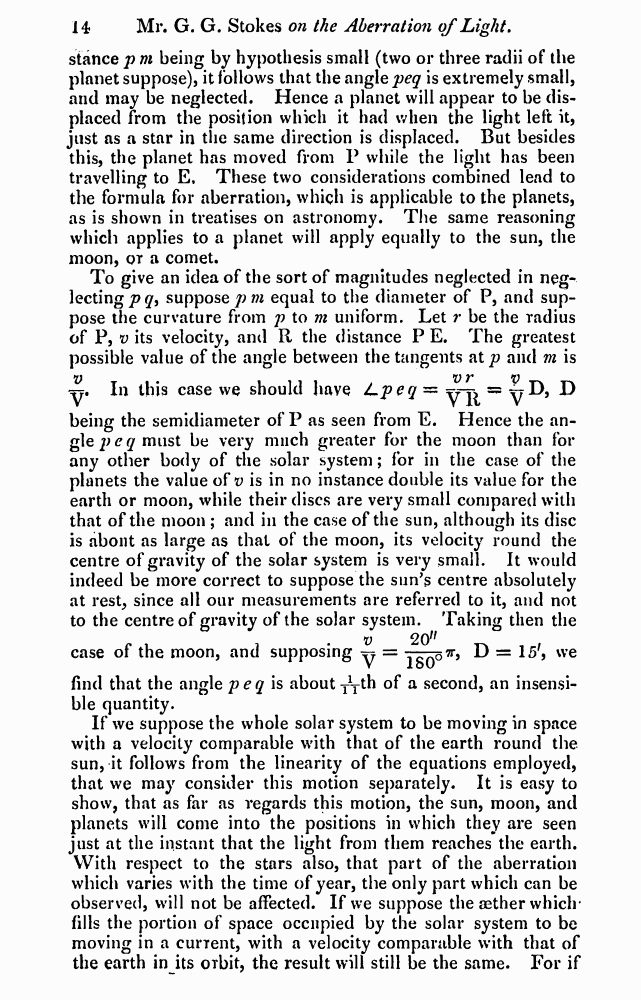﻿ G.G.Stokes "On the Aberration of Light" // Philosophical Magazine 1845, 27: Page 13

# G.G.Stokes "On the Aberration of Light" // Philosophical Magazine 1845, 27: Page 13

В начало   PDF, DjVu   <<<     Страница 13   >>>1  2  9  10  11  12  13 14  15 aether may be neglected, then to a point near the earth where we may still neglect the motion of the aether, and lastly to the point of the' earth’s surface at which the planet is viewed. For the first part we ghall have u% = 0, t>2 = 0, and vx will be the resolved parts of the planet’s velocity. The increments tt V of a and /3 for the first interval will be, therefore, — y, — ^. For the second interval a and /3 will remain constant, while 11 v for the third their increments will be y, y, just as in the case of a star, w2 and i>2 being now the resolved parts of the earth’s velocity. Fig. 1. P Fig. 1 represents what is conceived to take place. P is the planet in the position it had when the light quitted it; E the earth in the position it has when the light reaches it. The lines a b, cd, &c. represent a small portion of a wave of light in its successive positions. The arrows represent the directions in which P and E may be conceived to move. The breadth a b is supposed to be comparable to the breadth of a telescope. In fig. %pmne represents an orthogonal trajectory to the surfaces a b, cd, &c.; p is the point of the planet from which the light starts, e the point of the earth which it reaches. The trajectorypmnc may be considered a straight line, except near the ends p and e, where it will be a little curved, as from p to m and from e to n. The curvature at e will have the same effect on the apparent position of the planet as it would have on that of a star in the same direction : as to the curvature at p, if we draw jpy perpendicular to mn produced, the curvature will have the effect of causing p to be seen as if it were at q. Now the angle between the tangents at p and m being that through which a star in the direction of e is displaced by aberration to an observer at p, and the di- The lines towards P in fig. 1. should lean in the opposite direction. stance p m being by hypothesis small (two or three radii of the planet suppose), it follows that the angle peq is extremely small, and may be neglected. Hence a planet will appear to be displaced from the position which it had when the light left it, just as a star in the same direction is displaced. But besides this, the planet has moved from P while the light has been travelling to E, These two considerations combined lead to the formula for aberration, which is applicable to the planets, as is shown in treatises on astronomy. The same reasoning which applies to a planet will apply equally to the sun, the moon, or a comet. To give an idea of the sort of magnitudes neglected in neglecting p q, suppose pm equal to the diameter of P, and suppose the curvature from p to m uniform. Let r be the radius of P, v its velocity, and R the distance P E. The greatest possible value of the angle between the tangents at p and m is v v r v In this case we should have JLpeq = y-y^ = y ® being the semidiameter of P as seen from E. Hence the angle peq must be very much greater for the moon than for any other body of the solar system; for in the case of the planets the value of v is in no instance double its value for the earth or moon, while their discs are very small compared with that of the moon ; and in the case of the sun, although its disc is about as large as that of the moon, its velocity round the centre of gravity of the solar system is very small. It would indeed be more correct to suppose the sun’s centre absolutely at rest, since all our measurements are referred to it, and not to the centre of gravity of the solar system. Taking then the V 20lf case of the moon, and supposing y = - 7r, D = 15', we find that the angle peq is about yTth of a second, an insensible quantity. If we suppose the whole solar system to be moving in space with a velocity comparable with that of the earth round the sun, it follows from the linearity of the equations employed, that we may consider this motion separately. It is easy to show, that as far as regards this motion, the sun, moon, and planets will come into the positions in which they are seen just at the instant that the light from them reaches the earth. With respect to the stars also, that part of the aberration which varies with the time of year, the only part which can be observed, will not be affected. If we suppose the aether which* fills the portion of space occupied by the solar system to be moving in a current, with a velocity comparable with that of the earth in its oibit, the result will still be the same. For if